HistoryMathematiciansAll formulasNum. approx.SoftwaresMisc. math.DigitsPoetryPapers/videosDelirium !Pi-DayImagesMusicLinksBibliography Boris Gourévitch The world of Pi - V2.57 modif. 13/04/2013HomeVersion historyGuestbookWho I amSome pictures (fr)AcknowledgementsLast modificationsContact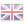Benoit Cloitre

## Calculation of the decimals of Pi: Some new with old stuff!

January 4, 2009

### To Sum up

By exploiting a simple geometric idea, Benoit, still as prolific, improves the efficients of Archimedes' method for the calculation of the decimals of. This approach mixes algorithms and series and allows in theory to obtain a convergence speed of as fast as we want it. The convergence stays for the moment linear.

### 1 The Formula

A modern version of Achimedes algorithms consiste of defining the algorithmand(1)

which allows to calculategiven that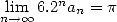(2)

The efficience of this method is not bad since the convergence is in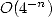. It is not extraordinary either and numerous other methods (algorithms, series...) also converges linearly, equlas it or are an improvement (without taking into account the algorithms by Salamin-Brent or the other Borwein's brother of course). We offer still to start from this old idea and to calculate the decimals ofwith a speed of how great we choose it to be (according to a pre calculation). Hence, for all values ofwe have the following formula which stay valid: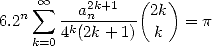(3)

### 2 Proof

We ask you to exactly calculate the strippy area below, which is a sector of a unit square.It is easy to see that this surface is worth(4)

By choosing, we have for allthe cercle divided into equal sectors and :(5)

because we find again the area of the unit circle. On the other hand, we have the well known series :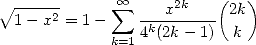(6)

i.e.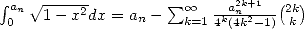and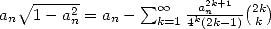and hence. The equation 5 hence becomes(7)

This gives a family of series converging as fast as we want towardsand hence improves Archimedes' algorithm .

### 3 Trials

For, if we calculate, then the serie(8)

gives however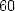good decimals ofat each term.